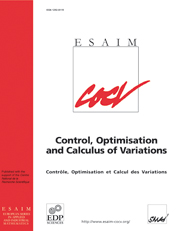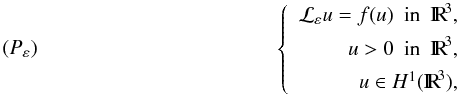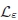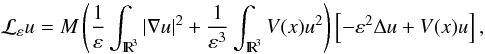Skip to main content Accessibility help
Home
Hostname: page-component-684bc48f8b-68png Total loading time: 0.4 Render date: 2021-04-12T17:02:09.709Z Has data issue: true Feature Flags: { "shouldUseShareProductTool": true, "shouldUseHypothesis": true, "isUnsiloEnabled": true, "metricsAbstractViews": false, "figures": false, "newCiteModal": false, "newCitedByModal": true }ESAIM: Control, Optimisation and Calculus of Variations

# Multiplicity and concentration behavior of positive solutions for a Schrödinger–Kirchhoff type problem via penalization method∗∗∗

Published online by Cambridge University Press:  03 March 2014

## Abstract

In this paper we are concerned with questions of multiplicity and concentration behavior of positive solutions of the elliptic problem

$$(P_{\var})\hspace*{4cm} \left\{ \begin{array}{rcl} \mathcal{L}_{\var}u=f(u) \ \ \mbox{in} \ \ \R^{3},\\[1.5mm] u>0 \ \ \mbox{in} \ \ \R^{3},\\[1.5mm] u \in H^{1}(\R^3), \end{array} \right.$$$\mathrm{\left(}{\mathit{P}}_{\mathit{\epsilon }}\mathrm{\right)}\left\{\begin{array}{c}\\ {\mathrm{ℒ}}_{\mathit{\epsilon }}\mathit{u}\mathrm{=}\mathit{f}\mathrm{\left(}\mathit{u}\mathrm{\right)}\mathrm{in}{\mathrm{IR}}^{\mathrm{3}}\mathit{,}\\ \mathit{u}\mathit{>}\mathrm{0}\mathrm{in}{\mathrm{IR}}^{\mathrm{3}}\mathit{,}\\ \mathit{u}\mathrm{\in }{\mathit{H}}^{\mathrm{1}}\mathrm{\left(}{\mathrm{IR}}^{\mathrm{3}}\mathrm{\right)}\mathit{,}\end{array}\right\$

where ε is a small positive parameter, f : ℝ → ℝ is a continuous function, $$\mathcal{L}_{\var}$$${\mathrm{ℒ}}_{\mathit{\epsilon }}$ is a nonlocal operator defined by

$$\mathcal{L}_{\var}u=M\left(\dis\frac{1}{\var}\int_{\R^{3}}|\nabla u|^{2}+\frac{1}{\var^{3}}\dis\int_{\R^{3}}V(x)u^{2}\right)\left[-\var^{2}\Delta u + V(x)u \right],$$${\mathrm{ℒ}}_{\mathit{\epsilon }}\mathit{u}\mathrm{=}\mathit{M}\left(\frac{\mathrm{1}}{\mathit{\epsilon }}{\mathrm{\int }}_{{\mathrm{IR}}^{\mathrm{3}}}\mathrm{|}\mathrm{\nabla }\mathit{u}{\mathrm{|}}^{\mathrm{2}}\mathrm{+}\frac{\mathrm{1}}{{\mathit{\epsilon }}^{\mathrm{3}}}{\mathrm{\int }}_{{\mathrm{IR}}^{\mathrm{3}}}\mathit{V}\mathrm{\left(}\mathit{x}\mathrm{\right)}{\mathit{u}}^{\mathrm{2}}\right)\mathrm{\left[}\mathrm{-}{\mathit{\epsilon }}^{\mathrm{2}}\mathrm{\Delta }\mathit{u}\mathrm{+}\mathit{V}\mathrm{\left(}\mathit{x}\mathrm{\right)}{\mathit{u}}^{\mathrm{\right]}}\mathit{,}$

M : IR+ → IR+ and V : IR3 → IR are continuous functions which verify some hypotheses.

## Keywords

Type
Research Article
Information
Copyright
© EDP Sciences, SMAI, 2014

## Access options

Get access to the full version of this content by using one of the access options below.

## References

Alves, C.O. and Corrêa, F.J.S.A., On existence of solutions for a class of problem involving a nonlinear operator. Commun. Appl. Nonlinear Anal. 8 (2001) 4356. Google Scholar
Alves, C.O., Corrêa, F.J.S.A. and Figueiredo, G.M., On a class of nonlocal elliptic problems with critical growth. Differ. Equ. Appl. 2 (2010) 409417. Google Scholar
Alves, C.O., Corrêa, F.J.S.A. and Ma, T.F., Positive solutions for a quasilinear elliptic equation of Kirchhoff type. Comput. Math. Appl. 49 (2005) 8593. Google Scholar
Alves, C.O. and Figueiredo, G.M., Nonlinear perturbations of a periodic Kirchhoff equation in IRN. Nonlinear Anal. 75 (2012) 27502759. Google Scholar
Alves, C.O. and Figueiredo, G.M., Multiplicity of positive solutions for a quasilinear problem in IRN via penalization method. Adv. Nonlinear Stud. 5 (2005) 551572. Google Scholar
Alves, C.O., Figueiredo, G.M. and Furtado, M.F., Multiple solutions for a Nonlinear Schrödinger Equation with Magnetic Fields. Commun. Partial Differ. Equ. 36 (2011) 122. Google Scholar
Ambrosetti, A., Badiale, M. and Cingolani, S., Semiclassical stats of nonlinear Schrodinger equations with potentials. Arch. Ration. Mech. Anal. 140 (1997) 285300. Google Scholar
Ambrosetti, A., Malchiodi, A. and Secchi, S., Multiplicity results for some nonlinear Schorodinger equations with potentials. Arch. Ration. Mech. Anal. 159 (2001) 253271. Google Scholar
Anelo, G., A uniqueness result for a nonlocal equation of Kirchhoff equation type and some related open problem. J. Math. Anal. Appl. 373 (2011) 248251. Google Scholar
G. Anelo, On a pertubed Dirichlet problem for a nonlocal differential equation of Kirchhoff type. BVP (2011) 891430.
Cingolani, S. and Lazzo, M., Multiple positive solutions to nonlinear Schrodinger equations with competing potential functions. J. Differ. Equ. 160 (2000) 118138. Google Scholar
Del Pino, M. and Felmer, P.L., Local Mountain Pass for semilinear elliptic problems in unbounded domains. Calc. Var. 4 (1996) 121137. Google Scholar
Ekeland, I., On the variational principle. J. Math. Anal. Appl. 47 (1974) 324353. Google Scholar
Figueiredo, G.M. and Santos Junior, J.R., Multiplicity of solutions for a Kirchhoff equation with subcritical or critical growth. Differ. Integral Equ. 25 (2012) 853868. Google Scholar
Floer, A. and Weinstein, A., Nonspreading wave packets for the cubic Schrodinger equation with a bounded potential. J. Funct. Anal. 69 (1986) 397408. Google Scholar
He, X. and Zou, W., Existence and concentration of positive solutions for a Kirchhoff equation in IR3. J. Differ. Equ. 252 (2012) 18131834. Google Scholar
G. Kirchhoff, Mechanik. Teubner, Leipzig (1883).
Li, Y., Li, F. and Shi, J., Existence of a positive solution to Kirchhoff type problems without compactness conditions. J. Differ. Equ. 253 (2012) 22852294. Google Scholar
Li, G., Some properties of weak solutions of nonlinear scalar field equations. Ann. Acad. Sci. Fenincae Ser. A 14 (1989) 2736. Google Scholar
J.L. Lions, On some questions in boundary value problems of mathematical physics International Symposium on Continuum, Mech. Partial Differ. Equ. Rio de Janeiro(1977). In vol. 30 of Math. Stud. North-Holland, Amsterdam (1978) 284–346.
Ma, T.F., Remarks on an elliptic equation of Kirchhoff type. Nonlinear Anal. 63 (2005) 19671977. Google Scholar
Moser, J., A new proof de Giorgi’s theorem concerning the regularity problem for elliptic differential equations. Commun. Pure Appl. Math. 13 (1960) 457468. Google Scholar
Nie, Jianjun and Wu, Xian, Existence and multiplicity of non-trivial solutions for Schródinger–Kirchhoff equations with radial potential. Nonlinear Analysis 75 (2012) 34703479. Google Scholar
Rabinowitz, P.H., On a class of nonlinear Schrodinger equations. Z. Angew Math. Phys. 43 (1992) 2742. Google Scholar
Szulkin, A. and Weth, T., Ground state solutions for some indefinite variational problems. J. Funct. Anal. 257 (2009) 38023822. Google Scholar
A. Szulkin and T. Weth, The method of Nehari manifold, Handbook of Nonconvex Analysis and Applications, edited by D.Y. Gao and D. Montreanu. International Press, Boston (2010) 597–632.
Wang, J., Tian, L., Xu, J. and Zhang, F., Multiplicity and concentration of positive solutions for a Kirchhoff type problem with critical growth. J. Differ. Equ. 253 (2012) 23142351. Google Scholar
M. Willem, Minimax Theorems. Birkhauser (1996).
Wu, Xian, Existence of nontrivial solutions and high energy solutions for Schrödinger–Kirchhoff-type equations in RN. Nonlinear Anal. RWA 12 (2011) 12781287. Google Scholar

### Full text views

Full text views reflects PDF downloads, PDFs sent to Google Drive, Dropbox and Kindle and HTML full text views.

Total number of HTML views: 0
Total number of PDF views: 26 *
View data table for this chart

* Views captured on Cambridge Core between September 2016 - 12th April 2021. This data will be updated every 24 hours.

# Send article to Kindle

To send this article to your Kindle, first ensure no-reply@cambridge.org is added to your Approved Personal Document E-mail List under your Personal Document Settings on the Manage Your Content and Devices page of your Amazon account. Then enter the ‘name’ part of your Kindle email address below. Find out more about sending to your Kindle. Find out more about sending to your Kindle.

Note you can select to send to either the @free.kindle.com or @kindle.com variations. ‘@free.kindle.com’ emails are free but can only be sent to your device when it is connected to wi-fi. ‘@kindle.com’ emails can be delivered even when you are not connected to wi-fi, but note that service fees apply.

Find out more about the Kindle Personal Document Service.

Multiplicity and concentration behavior of positive solutions for a Schrödinger–Kirchhoff type problem via penalization method∗∗
Available formats
×

# Send article to Dropbox

To send this article to your Dropbox account, please select one or more formats and confirm that you agree to abide by our usage policies. If this is the first time you use this feature, you will be asked to authorise Cambridge Core to connect with your <service> account. Find out more about sending content to Dropbox.

Multiplicity and concentration behavior of positive solutions for a Schrödinger–Kirchhoff type problem via penalization method∗∗
Available formats
×

# Send article to Google Drive

To send this article to your Google Drive account, please select one or more formats and confirm that you agree to abide by our usage policies. If this is the first time you use this feature, you will be asked to authorise Cambridge Core to connect with your <service> account. Find out more about sending content to Google Drive.

Multiplicity and concentration behavior of positive solutions for a Schrödinger–Kirchhoff type problem via penalization method∗∗
Available formats
×
×

#### Conflicting interests

Do you have any conflicting interests? *